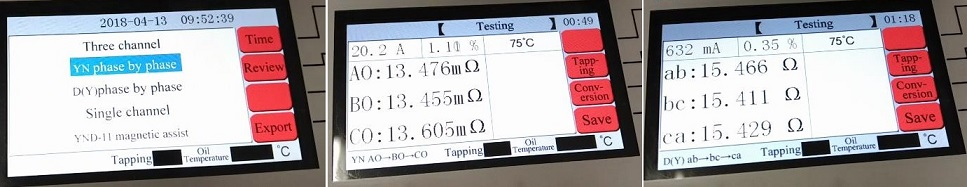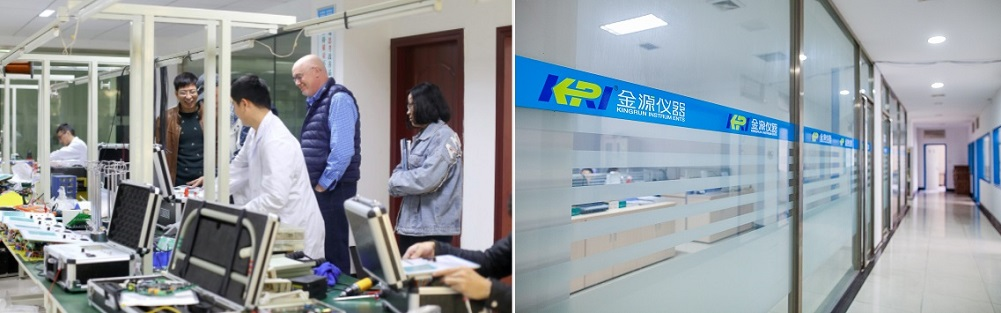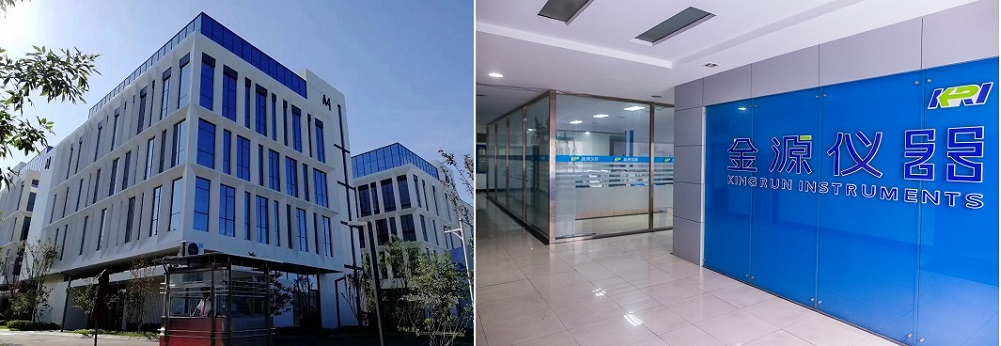News

# How to Calculate the Transformer DC Resistance Imbalance of the Transformer?

Causes and hazards of imbalanced transformer resistance

The imbalanced three-phase resistance load of the transformer will cause the three-phase operation to be unsymmetrical, resulting in zero-sequence current. On the other hand, the loss of the transformer will increase, and on the other hand, the effective capacity of the transformer will be reduced. The above two situations will lead to overheating of the transformer and aging of the insulating oil, which will reduce the insulation level of the winding, and will eventually lead to the damage of the transformer.
The main reasons for the imbalanced three-phase resistance of the transformer are as follows:

1. The contact of the tap changer is not in good contact. There may be problems such as oxidation and ablation of the contact, insufficient contact pressure of the contact, and uncleanness of the contact. Generally, the switch can be operated for a few more cycles to test again.
2. There is poor contact between the transformer winding and the lead wire, the lead wire and the bushing, the test clip and other connections or welding parts. It is recommended to carefully check the test wiring.
3. The windings are wound in multiple strands, and one or several strands are de-soldered.
4. The winding is partially damaged or short-circuited.# How to calculate the resistance imbalance rate of transformer?

Dividing the maximum number of three-phase subtracted by the three-phase average is the unbalance rate.

Such as OA=5.761, OB=5.772, OC=5.758

DC resistance unbalance rate = (OB-OC) / ((OA + OA + OC) / 3) = (5.772-5.758) / ((5.761 + 5.772 + 5.758) / 3) = 0.014 / 5.7636667 = 0.002429 * 100% =0.2429

Imbalance: It means that the three-phase current (or voltage) amplitude is inconsistent in the power system, and the amplitude difference exceeds the specified range.

According to the power transformer with the specified capacity of 1. 6MVA or above, the phase resistance imbalance rate should not exceed 2%, the winding without neutral point, the line resistance unbalance rate should not exceed 1%; the capacity of 1.6MVA and below Transformer, the phase resistance unbalance rate is generally not more than 4%, and the line resistance imbalance rate is generally not more than 2%.

From the engineering practical point of view, it can be considered that the line resistance imbalance is half of the phase resistance unbalance rate. Therefore, it is recommended that for all three-phase transformers, regardless of their capacity, only the unbalance rate limit of the DC line resistance can be specified as 2%.

JYR-20S DC winding resistance tester adopting simultaneous testing of three phases when Yn type is tested, high-voltage YN three-phase simultaneous measurement compensation method (including O-phase resistance),and calculate balance rate of resistance of three phases automatically.Please Click below Pictures for other Transformer DC Winding Testers from Kingrun: